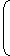# Time, Distance and Speed

Today we shall discuss another important topic of Aptitude - Time and Distance. In these type of problems you have to do problems on the speed of Train, Boat etc. Initially these problems may look little confusing, but if you practice well these are very interesting, easy to solve and help you to get good marks. But please keep in mind that the problems of this type are little confusing, people often lose marks because of their lack of concentration. They may feel that they've got the correct answer and choose the answer according to that, but they often endup forgetting CONVERSION (you will know what we've meant by this conversion later). Now before going into details, lets discuss some useful formulas of Time, Distance and Speed.

The first basic formula is, the formula of Distance...

Distance = Time X Speed

so obviously,

 Speed =Distanceand Time =DistanceTime Speed

Well, now lets talk about the units of expression. Normally, speed is expressed in kilometers per hour (kmph), distance in metres and time in seconds. Hence, one must remember the conversion factor of speed given below.

The above factor is obtained as follows,

1 kmph = 1 Km / 1 Hour  =  1000 m / 3600 Sec = 5/18 metre/sec (this is the key point in the problems of Time, Distance and Speed)

Thus , 18 kmph = 5 metre/sec

#### To convert the speed from metre/second to kmph., we divide it by 5/18 (or multiply it by 18/5)

• Here please keep in mind that due to the above factor, the speeds given in the questions of competitive exams are normally in multiples of 18, or at least in multiples of 9).

#### In case of speed - distance examples involving a train, the following possibilities exist.

• A train is crossing a Pole or a standing person
• A train is crossing a bridge, a platform or a tunnel etc.
• A train is crossing another train which is running in the opposite direction or in the same direction.

#### A train is crossing a pole or a standing person

• Here, the speed of the train and the length of the train are required to be known, in order to determine the time taken by the train to cross the pole/standing person. SInce a pole is stationary and the person is standing, the train is required to travel a distance equal to its own length. Thus, the application of the formula Distance = Speed x Time would directly give the solution.

#### A train is crossing a bridge, a platform or a tunnel etc.

• Here the speed of the train, the length of the train and the length of the bridge / platform / tunnel are required to be known, in order to determine the time taken by the train to cross the same completely. IN this case, the total distance to be traveled by the train would be the sum of its own length and the length of the bridge/platform.tunnel.
• The application of formula Distance = Speed x Time would then give the solution.

#### A train crossing another train running in the opposite direction

• Here the speeds and lengths of both the trains are required to be known, in order to determine the time required by the trains to cross each other. The total distance would be the sum of the lengths of both the trains whereas the effective speed of crossing would be the sum of the speeds of both the trains, since they are running in opposite directions.

#### A train is crossing another train running in the same direction.

• here, speeds and lengths of both the trains are required, in order to determine the time required by one of the trains (moving faster) to cross (overtake) the other train (moving slower). in this case, the total distance would be the sum of the lengths of both the trains whereas the effective speed of crossing (overtaking) would be the difference in the speeds of the trains. Thus it may be noted that if the speeds of both the trains are given to be equal, the trains would never cross (overtake) each other, since they are travelling in the same direction.

#### A boat travelling in a stream / river / still water.

• In case of a 'boat' travelling 'upstream' (against the current) or 'downstream' (alongwith the current), the speed of the boat (in still water), the speed o fthe current and the distance travelled are required to be know in order to determine the time required by the boat to travel that distance.
• In some examples, however, the distance travelled in both the cases (upstream and downstream) remains the same. thereby makin git possible to use the following formula :
(X + Y) x T1 = (X - Y) x T2 where
X = Speed of the boat in still water
Y = Speed of the current
T1 = Time taken by the boat to go downstream
T2 = Time taken by the boat to go upstream
Note that T1 < T2
Thats all for now friends. Prepare well these points. Tomorrow we shall discuss some problems on Time, Distance and Speed. Good Day :)

Liked this Post???
1.